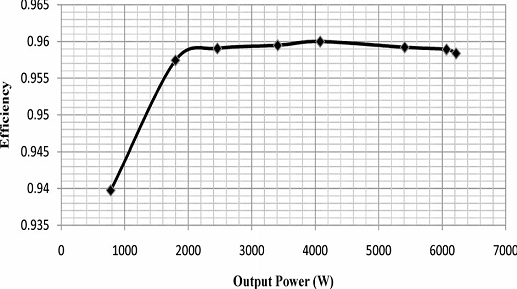Fixed Switching Frequency Sliding Mode Control for Single-Phase Unipolar Inverters

ABSTRACT:
Sliding mode control (SMC) is recognized as robust controller with a high stability in a wide range of operating conditions, although it suffers from chattering problem. In addition, it cannot be directly applied to multi switches power converters. In this paper, a high performance and fixed switching frequency sliding mode controller is proposed for a single-phase unipolar inverter. The chattering problem of SMC is eliminated by smoothing the control law in a narrow boundary layer, and a pulse width modulator produces the fixed frequency switching law for the inverter. The smoothing procedure is based on limitation of pulse width modulator. Although the smoothed control law limits the performance of SMC, regulation and dynamic response of the inverter output voltage are in an acceptable superior range. The performance of the proposed controller is verified by both simulation and experiments on a prototype 6-kVA inverter. The experimental results show that the total harmonic distortion of the output voltage is less than 1.1% and 1.7% at maximum linear and nonlinear load, respectively. Furthermore, the output dynamic performance of the inverter strictly conforms the standard IEC62040-3. Moreover, the measured efficiency of the inverter in the worst condition is better than 95.5%.
KEYWORDS:
1. Pulse width modulator
2. Sliding mode control
3. Unipolar single phase inverter

BLOCK DIAGRAM:Fig. 1. Proposed controller for single-phase inverters with a resonator in voltage loop.

EXPECTED SIMULATION RESULTS:Fig. 2. Simulation result. a) Output voltage and current at 6-kW linear load. b) Output voltage and current at 6-kVA nonlinear load with CF = 2.75 and PF = +0.7.Fig. 3. Simulation result: transient response of the output voltage for linear step load from zero to 100%Fig. 4. Simulation result: transient response of the output voltage for linear
step load from 100% to zero.Fig. 5. Experimental result: efficiency of inverter versus output power.

CONCLUSION:
In this paper, a fixed frequency SMC was presented for a single-phase inverter. The performance of the proposed controller has been demonstrated by a 6-kVA prototype. Experimental results show that the inverter is categorized in class1 of the IEC64020-3 standard for output dynamic performance. The inverter efficiency was measured up to 95.5% in the worst case.

Since the direct SMC cannot be applied to four switches unipolar inverter and it also suffers from the chattering problem, a PWM is employed to generate a fixed frequency switching law. The PWM modulates the smoothed discontinuous control law which is produced by SMC. To smooth the control law, the limitation of the PWM was considered.

The simulation and experimental results show that the load regulation is about 1% at the steady state as well. But, to obtain better regulation, a resonance compensator was added in the voltage loop. With this compensator, the load regulation was measured which has been below 0.2%.

REFERENCES:
 G. Venkataramanan and D.M. Divan, “Discrete time integral sliding mode control for discrete pulse modulated converters,” in Proc. 21st Annu. IEEE Power Electron. Spec. Conf., San Antonio, TX, 1990, pp. 67–73.
 J.Y.Hung,W. Gao, and J. C.Hung, “Variable structure control:Asurvey,” IEEE Trans. Ind. Electron., vol. 40, no. 1, pp. 2–22, Feb. 1993.
 E. Fossas and A. Ras, “Second order sliding mode control of a buck converter,” in Proc. 41st IEEE Conf. Decision Control, 2002, pp. 346– 347.
 C. Rech, H. Pinheiro, H. A. Gr¨undling, H. L. Hey, and J. R. Pinheiro, “A modified discrete control law for UPS applications,” IEEE Trans. Power Electron., vol. 18, no. 5, pp. 1138–1145, Sep. 2003.
 K. S. Low, K. L. Zhou, and D.W.Wang, “Digital odd harmonic repetitive control of a single- phase PWM inverter,” in Proc. 30th Annu. Conf. IEEE Ind. Electron. Soc., Busan, Korea, Nov. 2–6, 2004, pp. 6–11.